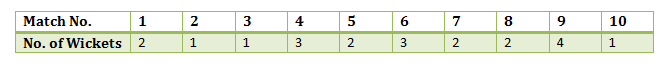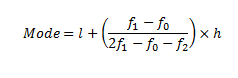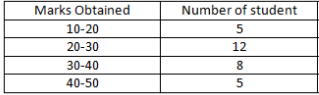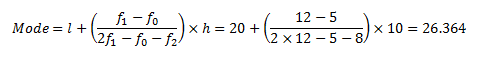# Mode

In statistics, the mode is the value that is repeatedly occurring in a given set. We can also say that the value or number in a data set, which has a high frequency or appears more frequently, is called mode or modal value. It is one of the three measures of central tendency, apart from mean and median. For example, the mode of the set {3, 7, 8, 8, 9}, is 8. Therefore, for a finite number of observations, we can easily find the mode. A set of values may have one mode or more than one mode or no mode at all.

In this article, you will understand the meaning of mode in statistics, formula for mode for grouped data and how to find the mode for the given data, i.e. for ungrouped and grouped data along with solved examples in detail.

## Mode Definition in Statistics

A mode is defined as the value that has a higher frequency in a given set of values. It is the value that appears the most number of times.

Example: In the given set of data: 2, 4, 5, 5, 6, 7, the mode of the data set is 5 since it has appeared in the set twice.

Statistics deals with the presentation, collection and analysis of data and information for a particular purpose. We use tables, graphs, pie charts, bar graphs, pictorial representation, etc. After the proper organization of the data, it must be further analyzed to infer helpful information.

For this purpose, frequently in statistics, we tend to represent a set of data by a representative value that roughly defines the entire data collection. This representative value is known as the measure of central tendency. By the name itself, it suggests that it is a value around which the data is centred. These measures of central tendency allow us to create a statistical summary of the vast, organized data. One such measure of central tendency is the mode of data.

## Bimodal, Trimodal & Multimodal (More than one mode)

• When there are two modes in a data set, then the set is called bimodal

For example, The mode of Set A = {2,2,2,3,4,4,5,5,5} is 2 and 5, because both 2 and 5 is repeated three times in the given set.

• When there are three modes in a data set, then the set is called trimodal

For example, the mode of set A = {2,2,2,3,4,4,5,5,5,7,8,8,8} is 2, 5 and 8

• When there are four or more modes in a data set, then the set is called multimodal

## Mode Formula in Statistics (Ungrouped Data)

The value occurring most frequently in a set of observations is its mode. In other words, the mode of data is the observation having the highest frequency in a set of data. There is a possibility that more than one observation has the same frequency, i.e. a data set could have more than one mode. In such a case, the set of data is said to be multimodal.

Let us look into an example to get a better insight.

Example: The following table represents the number of wickets taken by a bowler in 10 matches. Find the mode of the given set of data.It can be seen that 2 wickets were taken by the bowler frequently in different matches. Hence, the mode of the given data is 2.

## Mode Formula For Grouped Data

In the case of grouped frequency distribution, calculation of mode just by looking into the frequency is not possible. To determine the mode of data in such cases we calculate the modal class. Mode lies inside the modal class. The mode of data is given by the formula:Where,

l = lower limit of the modal class

h = size of the class interval

f1 = frequency of the modal class

f0 = frequency of the class preceding the modal class

f2 = frequency of the class succeeding the modal class

Let us take an example to understand this clearly.

## How to Find the Mode

Let us learn here how to find the mode of a given data with the help of examples.

Example 1: Find the mode of the given data set: 3, 3, 6, 9, 15, 15, 15, 27, 27, 37, 48.

Solution:  In the following list of numbers,

3, 3, 6, 9, 15, 15, 15, 27, 27, 37, 48

15 is the mode since it is appearing more number of times in the set compared to other numbers.

Example 2: Find the mode of 4, 4, 4, 9, 15, 15, 15, 27, 37, 48 data set.

Solution: Given: 4, 4, 4, 9, 15, 15, 15, 27, 37, 48  is the data set.

As we know, a data set or set of values can have more than one mode if more than one value occurs with equal frequency and number of time compared to the other values in the set.

Hence, here both the number 4 and 15 are modes of the set.

Example 3: Find the mode of 3, 6, 9, 16, 27, 37, 48.

Solution: If no value or number in a data set appears more than once, then the set has no mode.

Hence, for set 3, 6, 9, 16, 27, 37, 48, there is no mode available.

Example 4: In a class of 30 students marks obtained by students in mathematics out of 50 is tabulated as below. Calculate the mode of data given.Solution:

The maximum class frequency is 12 and the class interval corresponding to this frequency is 20 – 30. Thus, the modal class is 20 – 30.

Lower limit of the modal class (l) = 20

Size of the class interval (h) = 10

Frequency of the modal class (f1) = 12

Frequency of the class preceding the modal class (f0) = 5

Frequency of the class succeeding the modal class (f2)= 8

Substituting these values in the formula we get;## Mean Median Mode Comparison

 Mean Median Mode Mean is the average value that is equal to the ration of sum of values in a data set and total number of values. Mean = Sum of observations/Number of observations Median is the central value of given set of values when arranged in an order. Mode is the most repetitive value of a given set of values. For example, if we have set of values = 2,2,3,4,5, then; Mean = (2+2+3+4+5)/5 = 3.2 Median = 3 Mode = 2

### Mode Median Mean Formula

There exists an empirical relationship between mode, median and mean and this can be expressed using the formula:

Mode = 3 Median – 2 Mean

### Practice Problems

1. Find the mode of the following marks (out of 10) obtained by 20 students:
4, 6, 5, 9, 3, 2, 7, 7, 6, 5, 4, 9, 10, 10, 3, 4, 7, 6, 9, 9
2. Find the mode for the following data set.
41, 39, 48, 52, 46, 62, 54, 40, 96, 52, 98, 40, 42, 52, 60
3. Find the mode of the given distribution.
 Class Interval 10 – 25 25 – 40 40 – 55 55 – 70 70 – 85 85 – 100 Frequency 12 9 17 16 20 16

To know more about measures of central tendency and mode of data, download BYJU’S – The Learning App.

## Frequently Asked Questions – FAQs

Q1

### What is mode in statistics?

A mode, in statistics, is defined as the value that has higher frequency in a given set of values. It is the value that appears the most number of times.
Q2

### How to find mode for given set of values?

If we have a set of values equal to 33,44,55,55,66. Then the most repeated value in the given set is 55. Therefore, mode of the given set is 55.
Q3

### Can there be two modes in a given set of data?

Yes, there can be two modes in a given set of data. Such values are called bimodal.
Q4

### What is trimodal and multimodal mode?

If there are three modes in a data set, then it is called trimodal and if there are four or more than four modes then it is called multimodal mode.
Q5

### What is no mode condition?

If the given set of observations do not have any value that is repeated in the set, more than once, then it is said to be no mode.